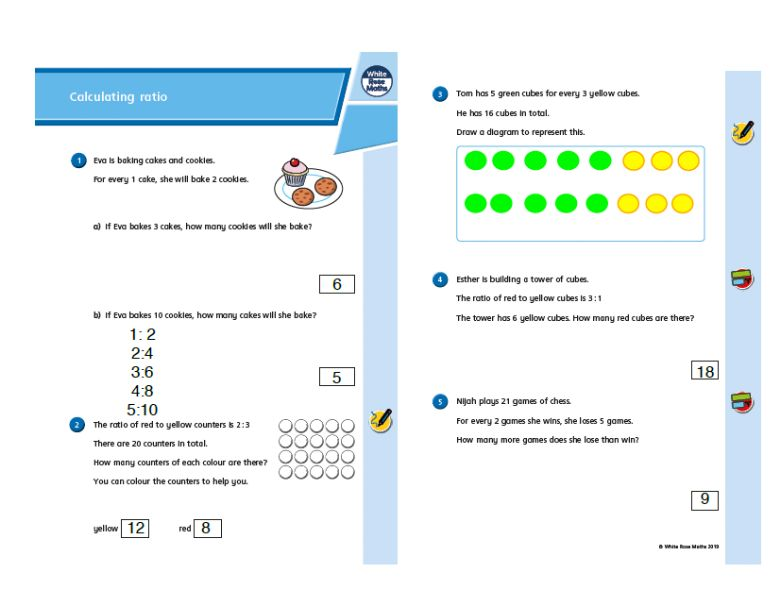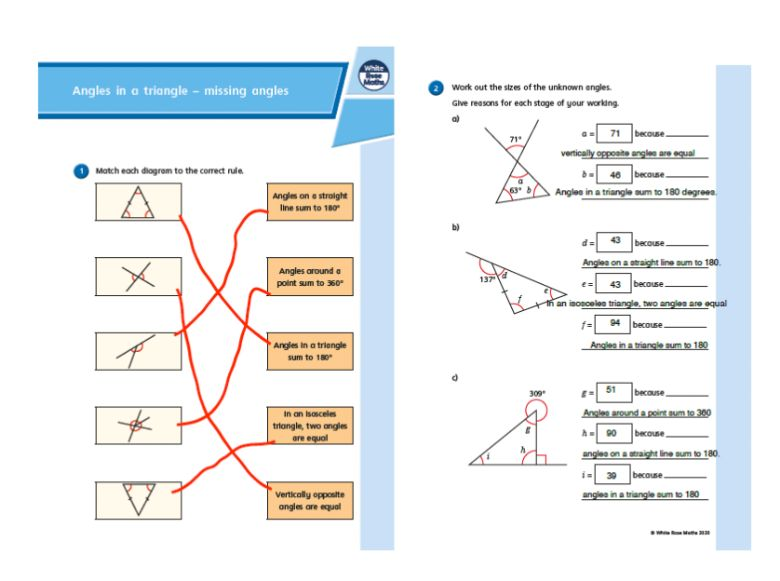# Summer Term: Maths 23/4/20 Ratio & Angles

Lesson 4 catch up: Calculating ratio AND lesson 4 actual: Angles in a triangle - missing angles

Instructions for lesson 4: 23/4/20

Fluency warm up: Times Tables Rock Stars

Catch up maths lesson: Week 1 lesson 4: Calculating ratio

* Watch the video tutorial;

* Get the Activity;

Actual maths lesson: Summer Term Week 1 (wk comm 20 April): Angles in a triangle - missing angles

* Watch the video tutorial;

* Get the Activity;

Let me know how you got on!

Here's L's work produced on 23/4/20. Beautifully presented using her ICT skills. Well done!